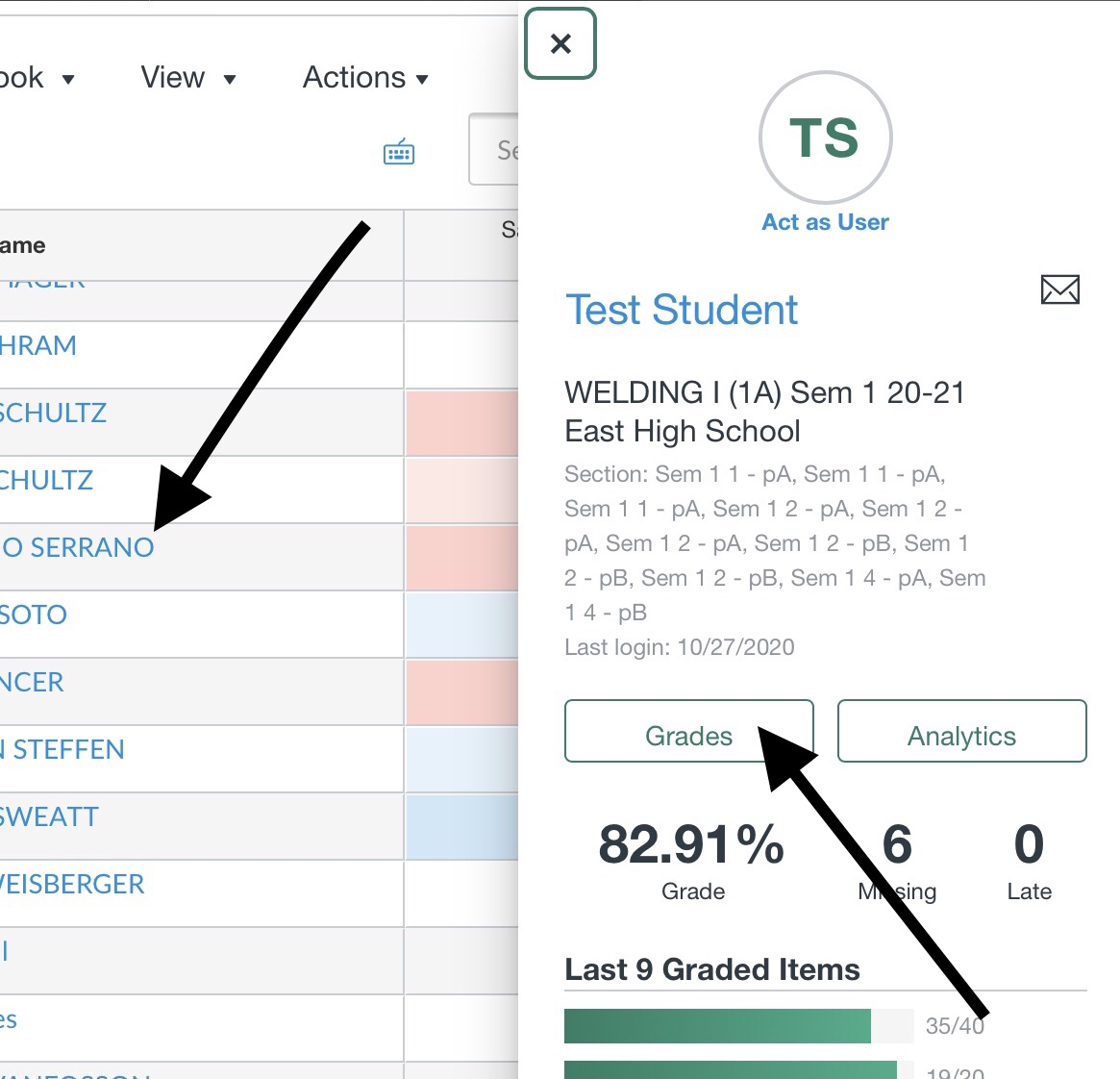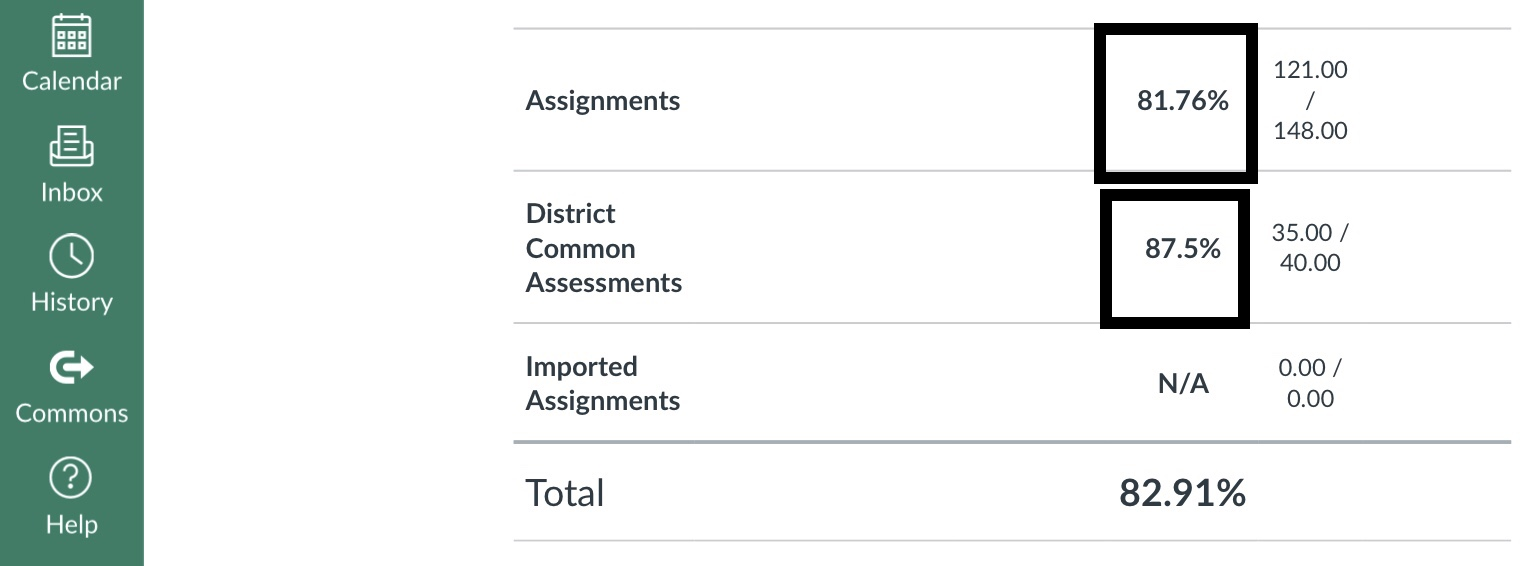Student grades have never automatically transferred from one course to another.    The only instance that this can occur is if the student was switched from one section to another with the same teacher AND the teacher already had the sections cross-listed in Canvas.

Teachers must do the following to manually transfer a student's grades:

1. Have the previous teacher export grades (1), then the new teacher would import grades (2).  However, the new teacher would need to do a 1-to-1 match for each Assignment grade into their own assignments by following these directions.
2. New teacher finds at the total number of points per Assignment Group currently in their gradebook.  Create an Assignment in each Assignment Group, called “Transfer Grade”, worth how many points that are currently in that Assignment Group and assign it to only the new student.  Look at the percentage for the Assignment Group the student had in the old class.  Calculate the Transfer Grade score the student needs to receive so that it equals the same percentage from the old class.  (example below)